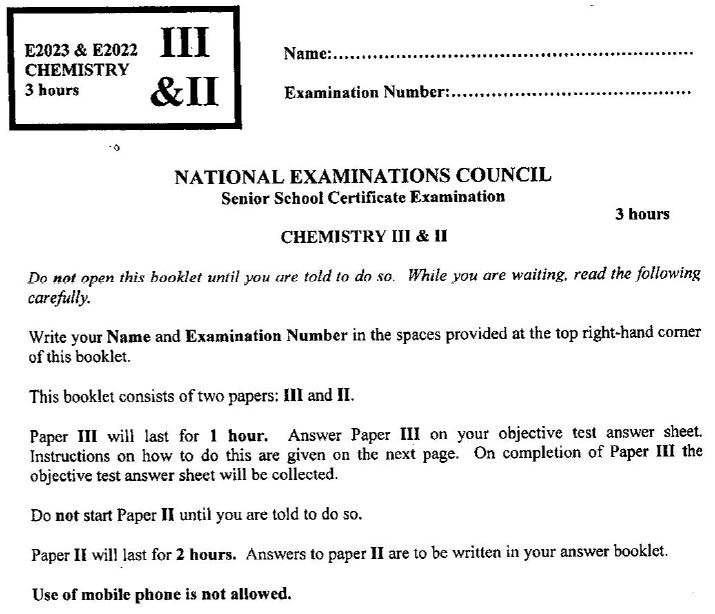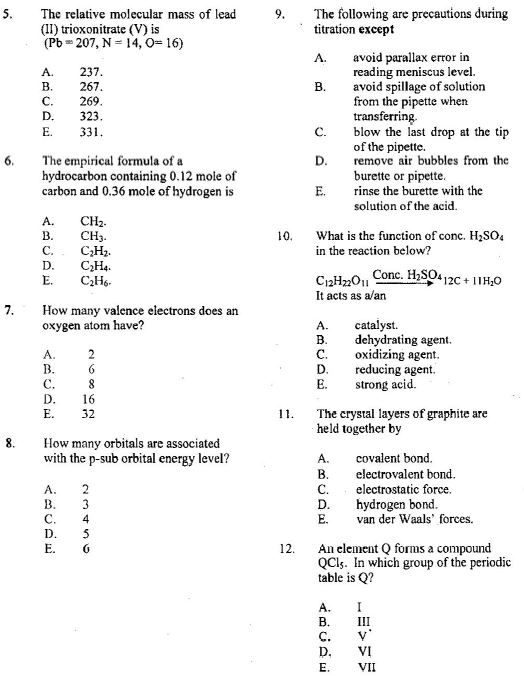# NECO Chemistry Questions & Answers 2021 (OBJ-Essay) Released

## See 2021 NECO Chemistry Answers & Questions Here.

The Neco chemistry answers for 2021 questions can now be seen here. The National Examination Council, NECO Chemistry SSCE paper is scheduled to be written on Tuesday 23rd November 2021 by 2:00 pm.

This NECO Chemistry questions paper is for Papers III & II: Objective & Essay and will take a total of 3hrs to write.

Here, we will be posting out the neco chemistry questions for candidates that will participate in the examination. Note that below is the questions from past questions and answers that we feel are likely questions for SSCE preparation.1. a) (i) What is the structure of the atom as proposed by Rutherford?
(ii) Distinguish between the atomic number and the mass number of an element.
(iii) Explain briefly why the relative atomic mass of chlorine is not a whole number.

b) (i) What is meant by first ionization energy?
(ii) List three properties of electrovalent compounds
(iii) Consider the following pairs of elements: 9F and 17CL; 12Mg and 20Ca.

2. a) (i) Define nuclear fission.
(ii) Consider the equilibrium reaction represented by the following equation: A2(g) + 3B2(g)   2AB3(g); H = + kJmol-1. Explain briefly the effect of each of the following changes on the equilibrium composition:

• increase in the concentration of B;
• decrease in pressure of the system;

b) The lattice energies of three sodium halides are as follows:

 Compound NaF NaBr NaI Lattice energy/kJmol-1 890 719 670

Explain briefly the trend.

c) State the property exhibited by nitrogen (IV) oxide in each of the following reactions:
(i) 4Cu + 2NO2        4CuO + N2;
(ii) H2O+ 2NO2       HNO3 + HNO2.

3. a) (i) Define saturated solution.
(ii) Distinguish between dative bond and covalent bond.
(iii) Explain why sugar and common salt do not conduct electricity in the solid state.
(iii) State the type of intermolecular forces present in: hydrogen fluoride; argon.
(iv) Consider the compounds with the following structures:
S – H —-N and 0 – H —–N
In which of the compounds is the hydrogen bond stronger? Give reason for your answer.

(b) (i) State Dalton’s Law of Partial Pressure.
(ii) If 200cm3 of carbon(IV) oxide were collected over water at 18°C and 700 mmHg, determine the volume of the dry gas at s.t.p.
[ standard vapour pressure of water at 18°C = 15 mmHg]

4. a) (i) Define ionic bond.
(ii) What type of bond(s) exist(s) in: magnesium oxide; ammonium ion?

b) (i) Determine the oxidation number of sulphur in Na2S2O3.
(iii) Give one example each of: acid salt; base salt.

c) (i) Name the type of energy change that occurs in each of the following processes;
I2(s)     ———>     I2(g);
C1(g) + e-      ——>     C1-(g).
(ii) State the effect of each of the following aqueous solutions on litmus paper:
Na2SO4(aq);
AlC13(aq)
(iii) Define the term efflorescence.
(iv) Give two uses of activated charcoal.

d) (i) State one use of each of the following processes in the chemical industry: hydrogenation of vegetable oil; cracking; esterification.
(ii) Calculate the amount of silver deposited in moles when 10920 coulombs of electricity is passed through a solution of a silver salt.
[Faraday constant = 96500 C mol-1]

5. a) (i) Define in terms of electron transfer
I.  oxidizing agent;
II.  reducing agent.
(ii) Write a balanced equation to show that carbon is a reducing agent.
(iii) State the change in oxidation number of the specie that reacted with carbon in 5 (a)(ii).

b) A gas X has a vapour density of 32. It reacts with sodium hydroxide solution to form salt and water only.  It decolourizes acidified potassium tetraoxomanganate (VII) solution and reacts with H2S to form sulphur.  Using the information provided:
identify gas X; state two properties exhibited by X; give two uses of X.

c) Consider the following substances:
(1) sodium;
(3) hydrogen;
(4) magnesium;
(5) oxygen.
Which of the substances
(i) conducts electricity?
(ii) is produced at the cathode during electrolysis of H2SO4(aq)?
(iii) corresponds to the molecular formula AB2  ?
(iv) is an alkaline earth metal?

d) (i) Define the term salt.
(ii) Mention two types of salt.
(iii) Give an example of each of the salts mentioned in 5(d)(ii) above.

e) In a neutralization reaction, dilute tetraoxosulphate (VI) acid completely reacted with sodium hydroxide solution.
(i) Write a balanced equation for the reaction.
(ii) How many moles of sodium hydroxide would be required for the complete neutralization of 0.50 moles of  tetraoxosulphate (VI) acid?

6.

Note: There is nothing like Neco chemistry Expo online. Neco Ssce candidates are to desist from patronizing online fraudsters / vendors who says they can provide such services as they are not real.

#### Neco Chemistry Objective Questions 2021.

1.

NOTE: That there is nothing like Neco chemistry expo online. Do not be deceived by fraudsters posing fake NECO chemistry answers on the internet.13.  At a particular temperature and pressure, 15.0 g of CO2 occupy 7.16 liters.  What is the volume of 12.0 g of CH4 at the same temperature and pressure?
A.

14.

Keep following this page. If you have any questions, endeavour to use the comment box below…

• I need the Neco 2021 questions and answers

Thanks

• Obj and theory answers chemistry pls

• Objective pls

• I loved to join this group,this website is great, thanks so much

• These chemistry questions above, the correct ones for neco 2021?

• I wish to joined dis group

• For chemistry obj n essay

• I just love unn-edu.info. I wrote Agric exams neco today main paper and can you imagine that some of the questions they posted here came out today . So I advise anything that is posted here should be given utmost attention.

• Thanks